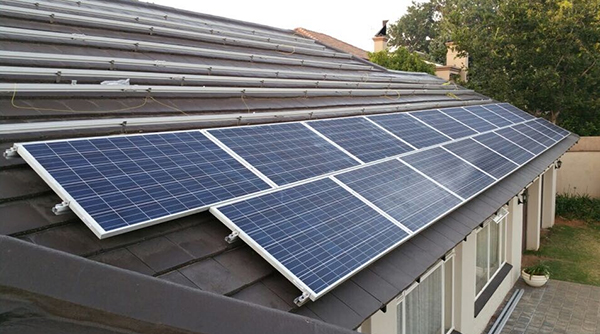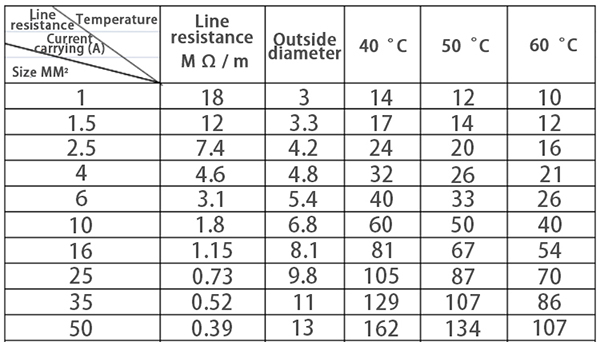# Where is the Best Installation Place for Solar Inverter?

If in the PV system, the component installation place is distant from the power grid connection place and the distance is over 300m, the solar inverter can be installed at three places. The first one is the place that is most approximate to the grid connection point, which can save the AC cable. The second one is the place that is closest to the component, which can save the DC cable. The third one is the middle place. Which place is the best choice?The most important factors for the PV system are the initial cost and power plant benefit. Thus, the benefits and disadvantages of the above mentioned three methods can be analyzed from these two aspects. Hereby a power station of 55kW can be analyzed. The component is installed at a place that is 300m away from the grid connection point and the component power is 280W. There are total 200 pieces of components and each 20 pieces are connected in series. Then, these 10 sets are connected with a 50kW grid tie solar inverter in parallel. There are total 10 groups of DC cable (20 wires) connecting the component and inverter. The AC output adopts the start connection method of 3W+N. The earth wire is connected nearby.

Cost comparison

Firstly, the cost analysis can be conducted. By far, the price of the general PV DC cable of PV 4mmis about 3.5 USD/m. The maximum current of the 60kW power station is 87A. The copper cable of 3*35+1*16 mm2 or 3*50+1*25 mm2 can be selected based on the distance. Now, the market price of 35mm2 is about 60 USD/m and the price of 50mm2 is about 90 USD/m. If the solar power inverter is close to the grid connection point, about 6000m (300*20=6000m) DC cable is required. In addition, about 10m 35mm2 AC cable will be needed. Thus, the total price of the DC and AC is 60*10+6000*3.5=21600 USD. If the solar inverter is installed at the place close to the component, then about 500m (25*20=500m) DC cable and about 300m 50mmAC cable will be used. Then, the total AC and DC cable price is 90*300+500*3.5=28750 USD. It can be seen that when the inverter is close to the grid connection point, the cost is lower.Efficiency comparison

Secondly, the efficiency can be compared. The solar power inverter line loss includes DC cable loss and the AC cable loss. DC line loss includes the DC cable loss and the MPPT matching loss. MPPT matching loss is related with the internal cable resistance and will be obvious when the cable is over 100m long. AC loss is the AC cable loss. The resistance of PV 4mm2 DC cable can be calculated as 4.6 mΩ/m and the resistance of the 35mmcopper cable can be calculated as 0.52 mΩ/m. The resistance of the 50mm2 copper cable can be counted as 0.39mΩ/m. DC current can be calculated as 8A and AC current can be calculated as 87A.

If the PV inverter is close to the grid connection point, the DC cable is about 300m long and large loss will be caused by the MPPT matching. The loss of the DC cable itself is about 1800W (P=6000*0.0046*82). The loss of the AC cable itself is about 118W (P=3*10*0.00052*872). If the MPPT matching loss is not counted, the total loss is 1918W. If the solar inverter is close to the component, about 500m DC cable is required and about 300m 50mmAC cable will be used. The loss of DC cable itself is about 150W (P=500*0.00046*82) and the loss of AC cable itself is about 2700W (P=3*300*0.00039*872). Thus, the total loss is about 2850W. It can be seen that when the PV inverter is close to the grid connection point, less loss will be caused.

In addition, when analyzing from the wire distribution aspect, it can be seen that about 20 pieces of thin DC wires are required and only one thick AC wire is used. Thus, the installation will be easier if the solar inverter is close to the component. As the DC does not pass zero point, the voltage is high. Thus, the DC will have higher danger in case of any fire disaster. Under this situation, it is safer to install the inverter at the place close to the component. If the PV system can not realize self-adoption, it needs to send to the grid and the AC cable shall be as short as possible.

Summary

Based on the calculation result, it can be seen that when the component is away from the grid connection point and the PV inverter is installed at the place close to the grid connection point, the initial cost and the energy loss will be lower but the difference is not very obvious. If the DC cable is over 100m long, the MPPT matching loss may be caused. In the actual installation, various conditions, including the cable distribution convenience and the power station safety, shall be considered.